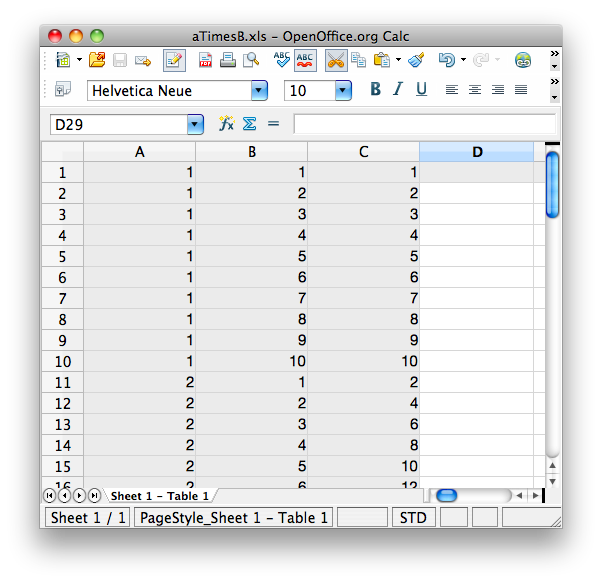# Data-driven tests With JUnit 4 and Excel

· Java Zone · Interview
Save
37.20K Views

One nice feature in JUnit 4 is that of Parameterized Tests, which let you do data-driven testing in JUnit with a minimum of fuss. It's easy enough, and very useful, to set up basic data-driven tests by defining your test data directly in your Java class. But what if you want to get your test data from somewhere else? In this article, we look at how to obtain test data from an Excel spreadsheet.

Parameterized tests allow data-driven tests in JUnit. That is, rather than having different of test cases that explore various aspects of your class's (or your application's) behavior, you define sets of input parameters and expected results, and test how your application (or, more often, one particular component) behaves. Data-driven tests are great for applications involving calculations, for testing ranges, boundary conditions and corner cases.

In JUnit, a typical parameterized test might look like this:

`@RunWith(Parameterized.class)public class PremiumTweetsServiceTest {    private int numberOfTweets;    private double expectedFee;    @Parameters    public static Collection data() {        return Arrays.asList(new Object[][] { { 0, 0.00 }, { 50, 5.00 },                { 99, 9.90 }, { 100, 10.00 }, { 101, 10.08 }, { 200, 18},                { 499, 41.92 }, { 500, 42 }, { 501, 42.05 }, { 1000, 67 },                { 10000, 517 }, });    }    public PremiumTweetsServiceTest(int numberOfTweets, double expectedFee) {        super();        this.numberOfTweets = numberOfTweets;        this.expectedFee = expectedFee;    }    @Test    public void shouldCalculateCorrectFee() {        PremiumTweetsService premiumTweetsService = new PremiumTweetsService();        double calculatedFees = premiumTweetsService.calculateFeesDue(numberOfTweets);        assertThat(calculatedFees, is(expectedFee));    }}`
The test class has member variables that correspond to input values (numberOfTweets) and expected results (expectedFee). The @RunWith(Parameterzed.class) annotation gets JUnit to inject your test data into instances of your test class, via the constructor.

The test data is provided by a method with the @Parameters annotation. This method needs to return a collection of arrays, but beyond that you can implement it however you want. In the above example, we just create an embedded array in the Java code. However, you can also get it from other sources. To illustrate this point, I wrote a simple class that reads in an Excel spreadsheet and provides the data in it in this form:

```@RunWith(Parameterized.class)

private double a;
private double b;
private double aTimesB;

@Parameters
public static Collection spreadsheetData() throws IOException {
}

super();
this.a = a;
this.b = b;
this.aTimesB = aTimesB;
}

@Test
public void shouldCalculateATimesB() {
double calculatedValue = a * b;
assertThat(calculatedValue, is(aTimesB));
}
}

```

The Excel spreadsheet contains multiplication tables in three columns:The SpreadsheetData class uses the Apache POI project to load data from an Excel spreadsheet and transform it into a list of Object arrays compatible with the @Parameters annotation. I've placed the source code, complete with unit-test examples on BitBucket. For the curious, the SpreadsheetData class is shown here:

```public class SpreadsheetData {

private transient Collection data = null;

public SpreadsheetData(final InputStream excelInputStream) throws IOException {
}

public Collection getData() {
return data;
}

throws IOException {
HSSFWorkbook workbook = new HSSFWorkbook(excelFile);

data = new ArrayList();
Sheet sheet = workbook.getSheetAt(0);

int numberOfColumns = countNonEmptyColumns(sheet);
List rows = new ArrayList();
List rowData = new ArrayList();

for (Row row : sheet) {
if (isEmpty(row)) {
break;
} else {
rowData.clear();
for (int column = 0; column < numberOfColumns; column++) {
Cell cell = row.getCell(column);
}
}
}
return rows;
}

private boolean isEmpty(final Row row) {
Cell firstCell = row.getCell(0);
boolean rowIsEmpty = (firstCell == null)
|| (firstCell.getCellType() == Cell.CELL_TYPE_BLANK);
return rowIsEmpty;
}

/**
* Count the number of columns, using the number of non-empty cells in the
* first row.
*/
private int countNonEmptyColumns(final Sheet sheet) {
Row firstRow = sheet.getRow(0);
return firstEmptyCellPosition(firstRow);
}

private int firstEmptyCellPosition(final Row cells) {
int columnCount = 0;
for (Cell cell : cells) {
if (cell.getCellType() == Cell.CELL_TYPE_BLANK) {
break;
}
columnCount++;
}
return columnCount;
}

private Object objectFrom(final HSSFWorkbook workbook, final Cell cell) {
Object cellValue = null;

if (cell.getCellType() == Cell.CELL_TYPE_STRING) {
cellValue = cell.getRichStringCellValue().getString();
} else if (cell.getCellType() == Cell.CELL_TYPE_NUMERIC) {
cellValue = getNumericCellValue(cell);
} else if (cell.getCellType() == Cell.CELL_TYPE_BOOLEAN) {
cellValue = cell.getBooleanCellValue();
} else if (cell.getCellType()  ==Cell.CELL_TYPE_FORMULA) {
cellValue = evaluateCellFormula(workbook, cell);
}

return cellValue;

}

private Object getNumericCellValue(final Cell cell) {
Object cellValue;
if (DateUtil.isCellDateFormatted(cell)) {
cellValue = new Date(cell.getDateCellValue().getTime());
} else {
cellValue = cell.getNumericCellValue();
}
return cellValue;
}

private Object evaluateCellFormula(final HSSFWorkbook workbook, final Cell cell) {
FormulaEvaluator evaluator = workbook.getCreationHelper()
.createFormulaEvaluator();
CellValue cellValue = evaluator.evaluate(cell);
Object result = null;

if (cellValue.getCellType() == Cell.CELL_TYPE_BOOLEAN) {
result = cellValue.getBooleanValue();
} else if (cellValue.getCellType() == Cell.CELL_TYPE_NUMERIC) {
result = cellValue.getNumberValue();
} else if (cellValue.getCellType() == Cell.CELL_TYPE_STRING) {
result = cellValue.getStringValue();
}

return result;
}
}

```

Data-driven testing is a great way to test calculation-based applications more thoroughly. In a real-world application, this Excel spreadsheet could be provided by the client or the end-user with the business logic encoded within the spreadsheet. (The POI library handles numerical calculations just fine, though it seems to have a bit of trouble with calculations using dates). In this scenario, the Excel spreadsheet becomes part of your acceptance tests, and helps to define your requirements, allows effective test-driven development of the code itself, and also acts as part of your acceptance tests.

From http://weblogs.java.net/blog/johnsmart

Topics:

Opinions expressed by DZone contributors are their own.